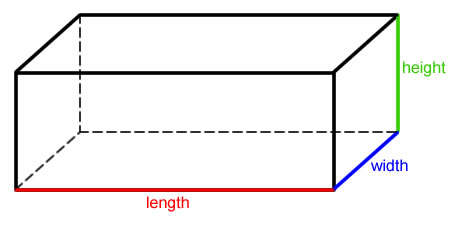# Rectangular prisms!

Algebra Level 4

Given a rectangular prism (as shown below) whose edge lengths have a sum of 36, what is the minimum value for the length of the line connecting two vertices which do not share a face?

If the minimum value can be expressed as $a\sqrt{b}$, where $a$ and $b$ are integers with $b$ square-free, submit your answer as $a+b$.×